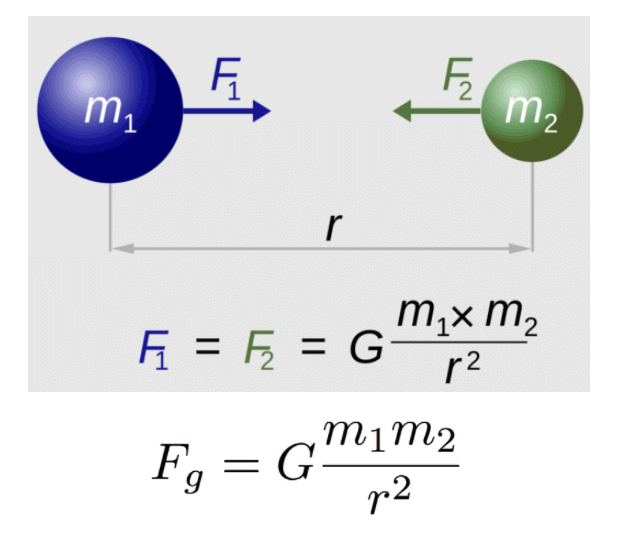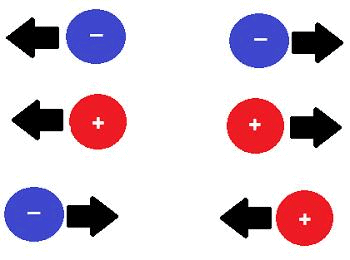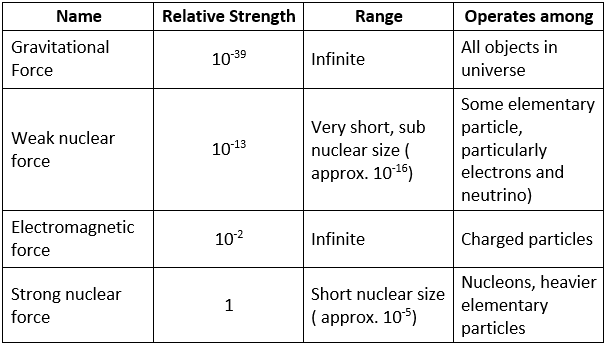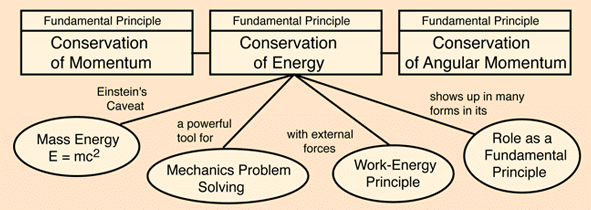NEET  >  Fundamental Forces in Nature

# Fundamental Forces in Nature - Notes | Study Physics Class 11 - NEET

 Table of contentsWhat are the Fundamental Forces?Types of Fundamental Forces in NatureConservation Laws in Physics1 Crore+ students have signed up on EduRev. Have you?

What are the Fundamental Forces?

The Fundamental forces, also known as Fundamental interaction, are the interactions that do not appear to be reducible to more basic interactions.

• There are four universal or fundamental forces in nature. Without these forces, all matter in the world will fall apart.
• Force as such is any pull or push that causes an object to alter its physical state (in terms of motion or deformity).• Newton defined a force as anything that causes an object of mass ‘m’ to move with an acceleration ‘a’.

Two principle types of approaches in physics are:

1. Unification: This approach considers all of the world's phenomena as a collection of universal laws in different domains and conditions.
Example: Law of gravitation applies both to an apple falling from a tree as well as the motion of planets around the sun. Electromagnetic Laws govern all electric and magnetic phenomena.
2. Reduction: This approach is to derive properties of a complex system from the properties and interaction of the constituent parts.
Example: Temperature studied under thermodynamics is also related to the average kinetic energy of molecules in the system (kinetic theory).

Types of Fundamental Forces in Nature

Following are the four fundamental forces in nature:

1. Gravitational force
2. Electromagnetic force
3. Strong nuclear force
4. Weak nuclear force

1. Gravitational Force

It stated that everybody in the universe, that has mass, attracts every other body with mass with a central force which is directly proportional to the product of their masses and inversely proportional to the square of the distance between them.

• It is the weakest of the four. It is also the attractive force that arises from the gravitational interaction.
• Gravitational Force is discovered by Sir Isaac Newton in 1687 in his Principia called Universal Law of Gravitation.Where,

• Fg is the gravitational force
• is called the Universal Gravitational Constant and its value is experimentally determined by Henry Cavendish to be 6.67 × 10-11 Nm2/kg2
• m1 and m2 are the masses of the objects in consideration
• r is the distance between the centres of the two objects in consideration

Have you wondered why is the universe not filled with floating human beings and cows and cars and other such things?

Obviously, because the gravitational force of the earth holds us to the planet. Gravitational force is the force responsible for holding planets in their orbits and this is possible only because of their infinitely long range.

2. Electromagnetic Force

• This is the second strongest force after the strong force and it acts on electrically charged particles. It has a strength of 1/137 relative to the strong force but has an infinite range.
• However, this force has both attractive and repulsive properties due to the two charges it possesses: Negative and Positive. Like charges repel while unlike charges attract and this can be demonstrated with a simple magnet or electric charges.Example of Electromagnetic Force

Did you know that you have never truly ever touched anything in the world?

You are matter, and we know that all matter is made up of atoms. Atoms in turn has a dense nucleus, protons, neutron and electrons.

And where are these electrons?

They occupy the outermost layer of an atom.

Now, what is the charge on electrons?

They have negative charges.

3. Strong Nuclear Force

• This force holds the nucleus of an atom together. It is the strongest of the forces, but it is very short-ranged.
• It acts over a range of about 10-15m, which is the average diameter of a medium-sized nucleus. This force is attractive.
• This is the most dominant force in reactions and is so strong that it binds protons with similar charges with the nucleus. It is mostly attractive in nature but can be repulsive sometimes. It has a very short range of just 1 fm.

4. Weak Nuclear Force

• This force is weak compared to the strong force as the name implies and has the shortest range of 10-18 m, which is 0.1% of the diameter of a proton.
• It is responsible for radioactive decay especially nuclear beta decay. All particles experience this force.Fundamental Forces of Nature

Unification of Forces
• It is a basic quest in physics. The electromagnetic and the weak nuclear forces have now been unified and are seen as aspects of a single ‘electro-weak force. Attempts are being made to unify electro-weak and the strong force.
• Conservation of energy-momentum angular momentum charge etc. are considered to be the fundamental laws in physics. Conservation laws have a deep connection with the symmetries of nature. Symmetries of space and time and other types of symmetries play a central role in modern theories of fundamental forces in nature.

Question for Fundamental Forces in Nature
Try yourself:The weakest of the four fundamental forces is:

Conservation Laws in Physics

• The physical quantities that remain unchanged in a process are called Conserved quantities.
• Some of the general conservation laws in nature include the laws of conservation of energy, mass, linear momentum, angular momentum, charge, parity, etc.• Some conservation laws are true for one fundamental force but not for the other. These conservation laws have a deep connection with the symmetries of nature.
• Symmetries of space and time and other types of symmetries play a central role in modern theories of fundamental forces in nature.

Question for Fundamental Forces in Nature
Try yourself: ________ can be either a force of attraction or repulsion while ____________ is only a force of attraction.

The document Fundamental Forces in Nature - Notes | Study Physics Class 11 - NEET is a part of the NEET Course Physics Class 11.
All you need of NEET at this link: NEET

## Physics Class 11

127 videos|464 docs|210 tests
 Use Code STAYHOME200 and get INR 200 additional OFF

## Physics Class 11

127 videos|464 docs|210 tests

### How to Prepare for NEET

Read our guide to prepare for NEET which is created by Toppers & the best Teachers

Track your progress, build streaks, highlight & save important lessons and more!

,

,

,

,

,

,

,

,

,

,

,

,

,

,

,

,

,

,

,

,

,

;